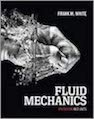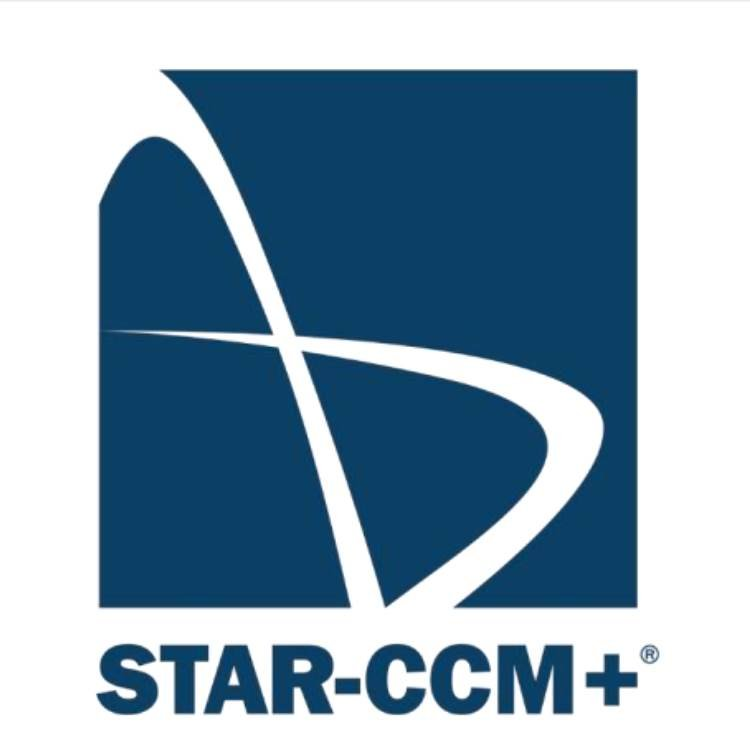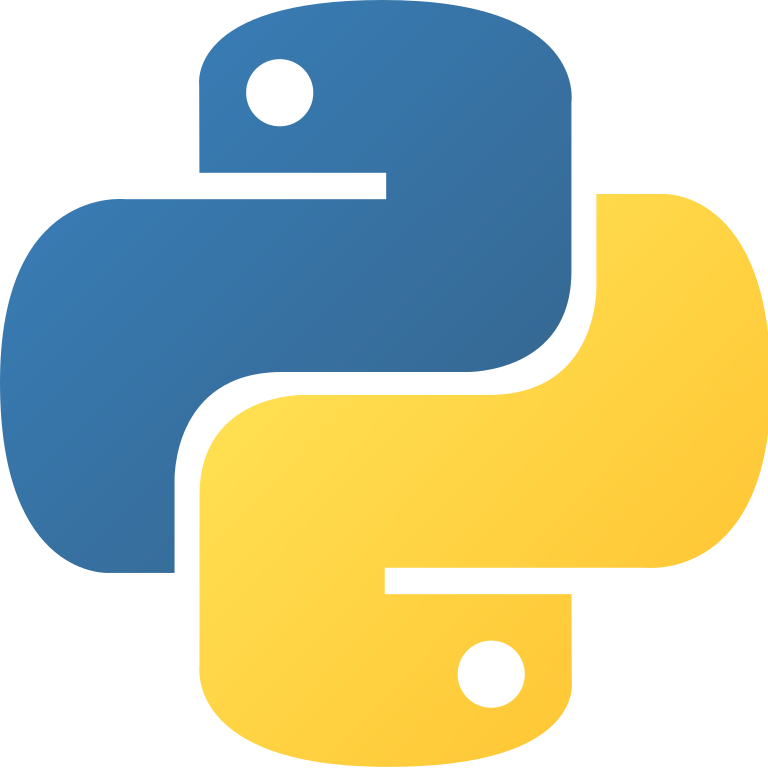Fluid Mechanics MTF053 Credits 7.5 ects (written exam 4.5, lab 1.5, computer assignments 1.5) Grading scale (written exam) fail, 3, 4, 5 Educational level Bachelor course Main field Mechanical Engineering Department Department of Mechanics and Maritime Sciences Division Division of Fluid Dynamics Language English Lectures In total 22 lectures + guest lectures Exerecises In total 17 exercises Labs & Assignments Two compulsory computer assignments and one hands-on lab

### Objectives

Gas and liquid flows are encountered in numerous engineering application and in many cases fluid mechanics plays a central role for the functionality. In fact, modern society with its dependence on fast ground and air transportation as well as reliable electricity generation would not function without fluid flow. The main objectives of the course are to convey to the students an overview of and familiarity with the field of fluid mechanics and the importance of this topic in the context of common engineering applications. This means that the student should acquire a general knowledge of the basic flow equations and how they are related to fundamental conservation principles and thermodynamic laws and relations. The concepts of turbulent flows and compressible flows will be introduced, and the aim is that the students should acquire the knowledge needed to be able to study courses dedicated to those subjects later. A general knowledge of, and some experience with, flow simulation software (Computational Fluid Dynamics (CFD) codes) should also be obtained after this course. The course makes a foundation for fluid related courses in, for example, the Applied Mechanics Master's programme, the Automotive Engineering Master's programme, the Sustainable Energy Systems Master's programme, and the Mobility Engineering Master's programme.

### Intended Learning Outcomes

After the completing the course, each student should be able to:

1. Explain the difference between a fluid and a solid in terms of forces and deformation
2. Understand and be able to explain the viscosity concept
3. Define the Reynolds number
4. Be able to categorize a flow and have knowledge about how to select applicable methods for the analysis of a specific flow based on category
5. Explain the difference between Lagrangian and Eulerian frame of reference and know when to use which approach
6. Explain what a boundary layer is and when/where/why it appears
7. Explain the concepts: streamline, pathline and streakline
8. Understand and be able to explain the concept shear stress
9. Explain how to do a force balance for fluid element (forces and pressure gradients)
10. Understand and explain buoyancy and cavitation
11. Solve problems involving hydrostatic pressure and buoyancy
12. Define Reynolds transport theorem using the concepts control volume and system
13. Derive the control volume formulation of the continuity, momentum, and energy equations using Reynolds transport theorem and solving problems using those relations
14. Derive the continuity, momentum and energy equations on differential form
15. Derive and use the Bernoulli equation (using the relation includes having knowledge about its limitations)
16. Understand and explain the concept Newtonian fluid
17. Explain how to use nondimensional numbers and the $$\Pi$$-theorem
18. Explain losses appearing in pipe flows
19. Explain the difference between laminar and turbulent pipe flow
20. Solve pipe flow problems using Moody charts
21. Explain how the flat plate boundary layer is developed (transition from laminar to turbulent flow)
22. Explain and use the Blasius equation
23. Define the Reynolds number for a flat plate boundary layer
24. Explain what is characteristic for a turbulent flow
25. Explain Reynolds decomposition and derive the RANS equations
26. Understand and explain the Boussinesq assumption and turbulent viscosity
27. Explain the difference between the regions in a boundary layer and what is characteristic for each of the regions (viscous sub layer, buffer region, log region)
28. Use von Kàrmàns integral relation
29. Explain flow separation (separated cylinder flow)
30. Explain how to delay or avoid separation
31. Derive the boundary layer formulation of the Navier-Stokes equations
32. Understand and explain displacement thickness and momentum thickness
33. Understand , explain and use the concepts drag, friction drag, pressure drag, and lift
34. Understand and explain how the shape and surface roughness of an object affects drag
35. Measure forces on an object in a flow
36. Define and explain vorticity
37. Understand and explain basic concepts of compressible flows (the gas law, speed of sound, Mach number, isentropic flow with changing area, normal shocks, oblique shocks, Prandtl-Meyer expansion)
38. Do a fluid flow simulation for as simple flow case using commercial Computational Fluid Dynamics (CFD) software

### Course LiteratureFluid Mechanics
Frank M. White
8th revised edition (in SI units)
McGraw-Hill 2016
ISBN: 978-9-814-72017-5

 Chapter 1 Introduction Chapter 2 Pressure Distribution in Fluids Chapter 3 Integral Relations for a Control Volume Chapter 4 Differential Relations for Fluid Flow Chapter 5 Dimensional Analysis and Similarity Chapter 6 Viscous Flow in Ducts Chapter 7 Flow Past Immersed Bodies Chapter 9 Compressible Flow

### Course Outline

In the course there are in total 22 lectures and 17 sessions with exercises. There is one compulsory hands-on fluid mechanics lab "Flow around immersed bodies" and two compulsory computer assignments; CA1 "Numerical analysis of fully developed channel flow" and CA2 "Numerical simulation of boundary layer flows".

The hands-on lab and the two assignments are done in groups (the same groups are used for all three compulsory course elements).### Examination

Of the total 7.5 ects, 4.5 ects are awarded if passing a written exam, 1.5 ects for the hands-on lab, and 1.5 ects for the two assignments (CA1 and CA2). The three parts are reported separately in Ladok.

The written exam consists of 8 problems each of which may give 10 points, i.e. in total 80 points.

Grades for the course will be given as follows:

 grade 3 4 5 number of points on the exam $$(p)$$ $$24 \le p < 36$$ $$36 \le p < 48$$ $$48 \le p$$